# ML Aggarwal Solutions for Class 10 Maths Chapter 2 Banking

ML Aggarwal Solutions for Class 10 Maths Chapter 2 Banking can be used by the students to understand the topics covered in an effective way. The solutions contain brief stepwise explanations, which are purely based on the latest syllabus of the ICSE board. To know more about these concepts, students can access ML Aggarwal Solutions for Class 10 Maths Banking PDF, from the links which are given below.

ML Aggarwal Solutions for Class 10 Maths Chapter 2 Banking has problems based on determining the maturity value, rate of interest, deposit, and important concepts, as per the prescribed textbook. By solving the problems on a regular basis, students can improve their conceptual knowledge, which is important from the exam perspective. The ML Aggarwal Solutions can be referred to as cross-check answers and understand the other ways of solving problems effortlessly.

## ML Aggarwal Solutions for Class 10 Maths Chapter 2 Banking :-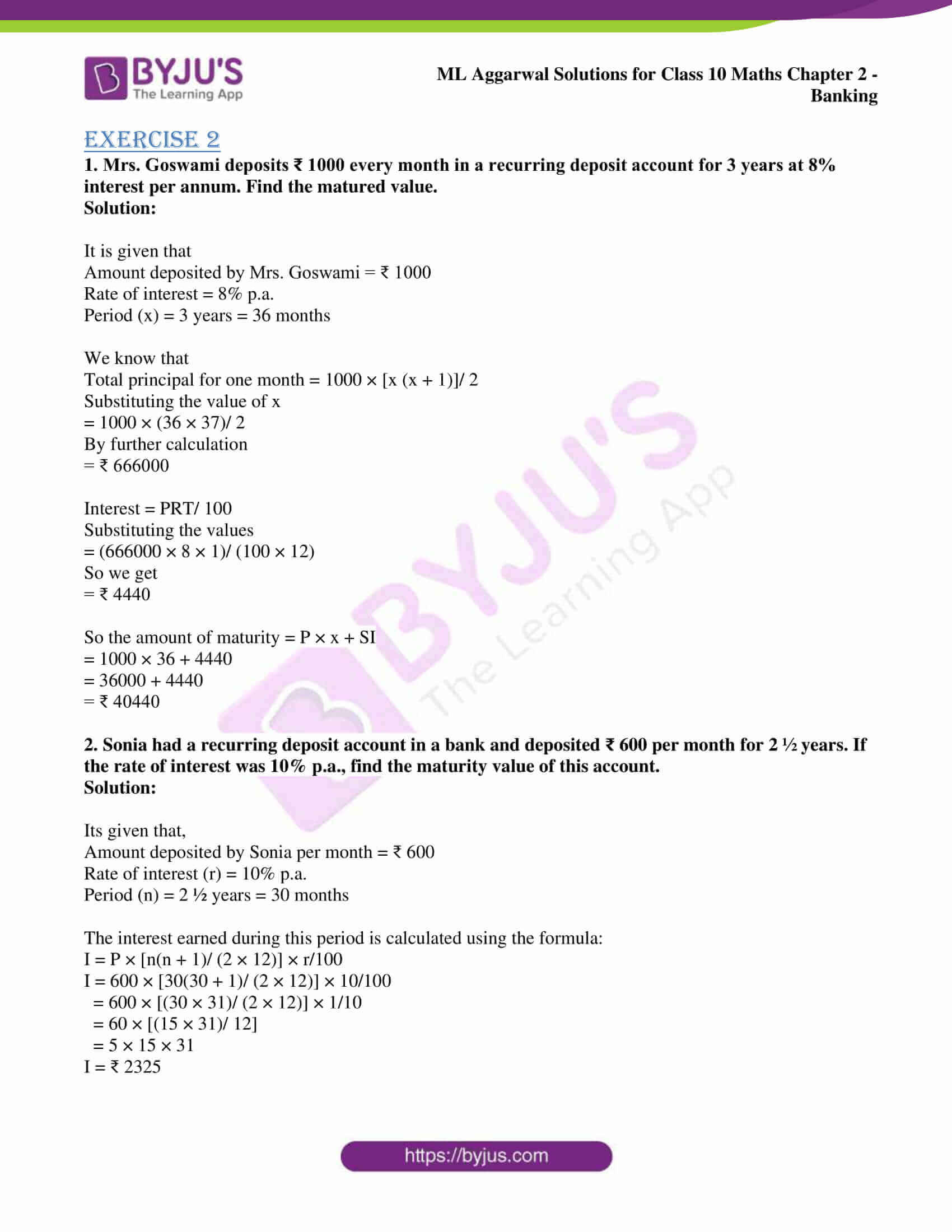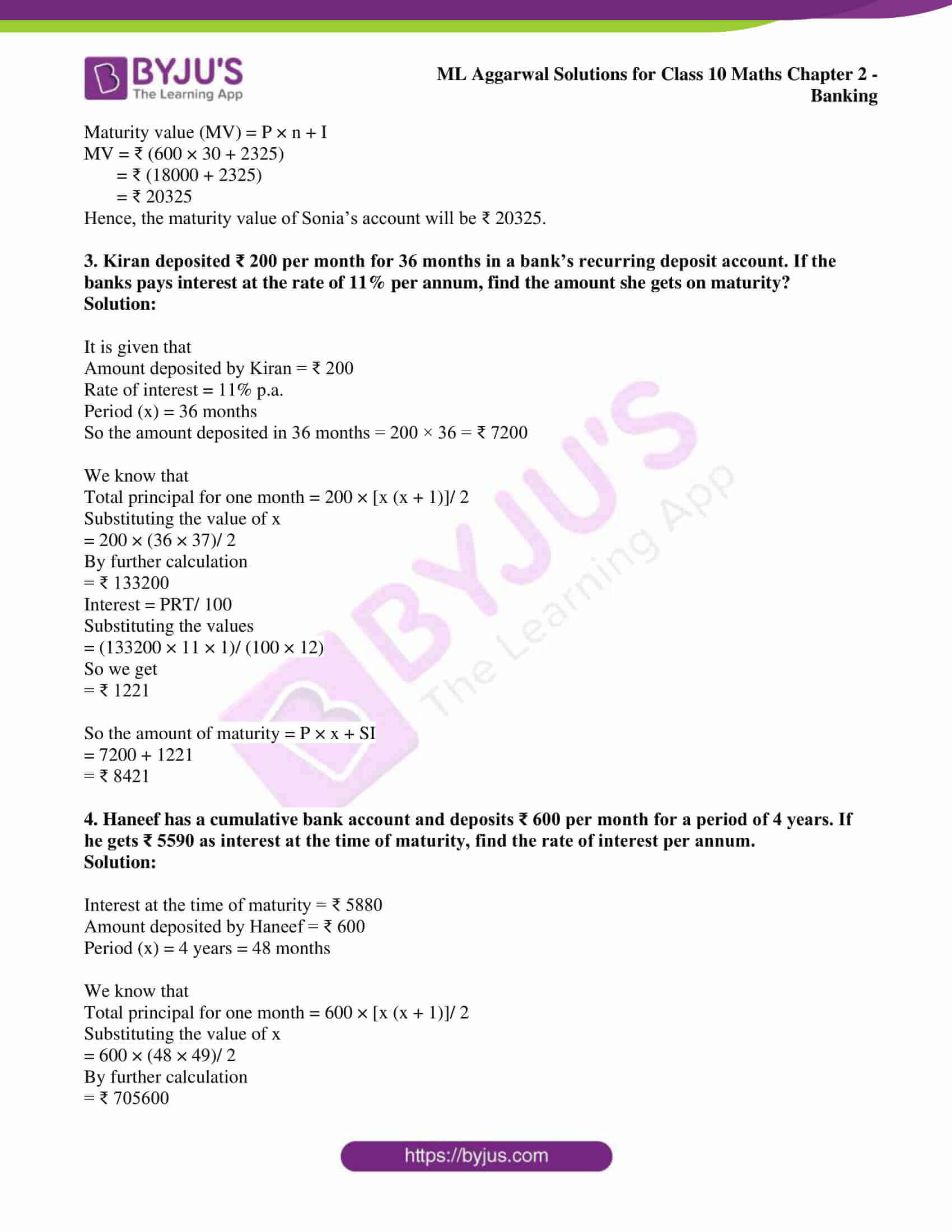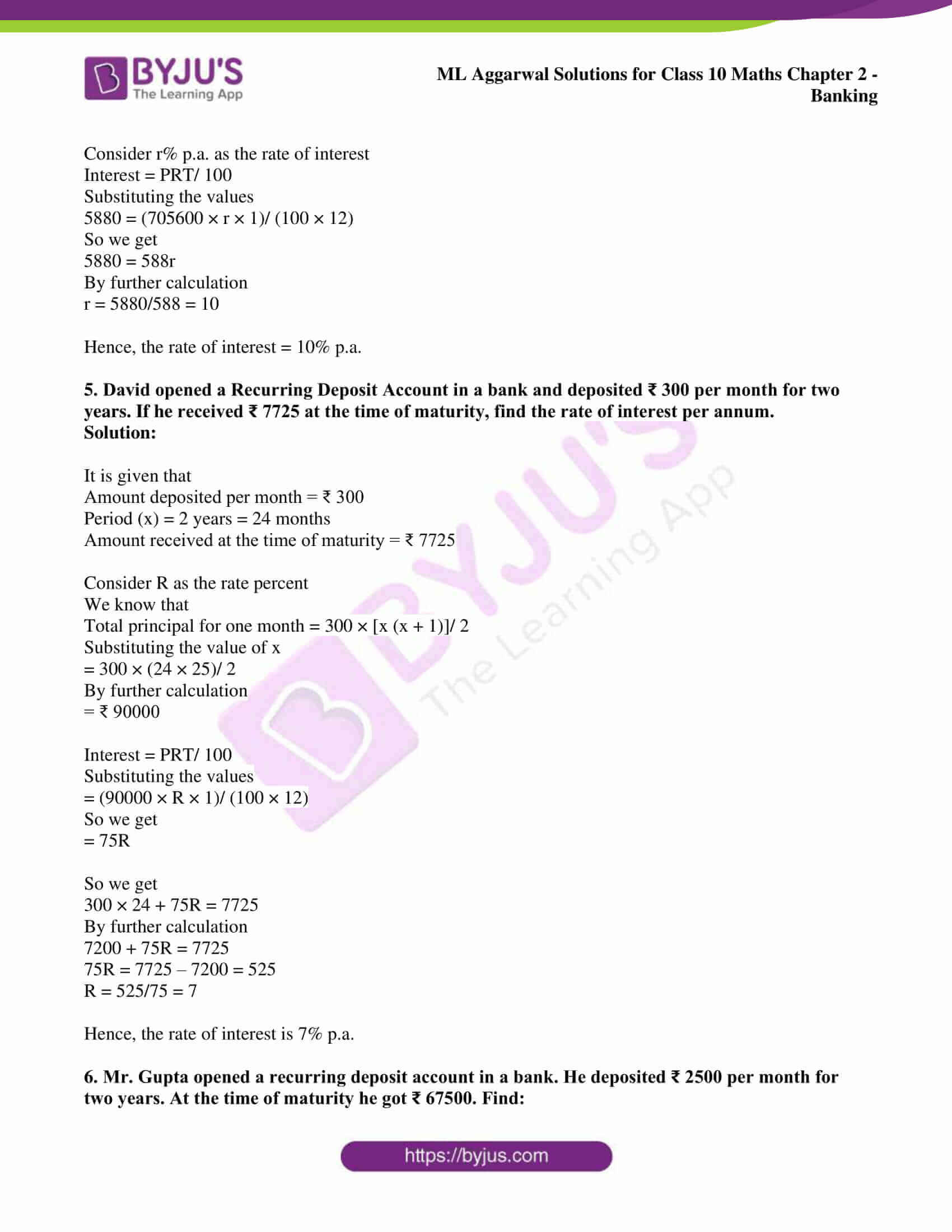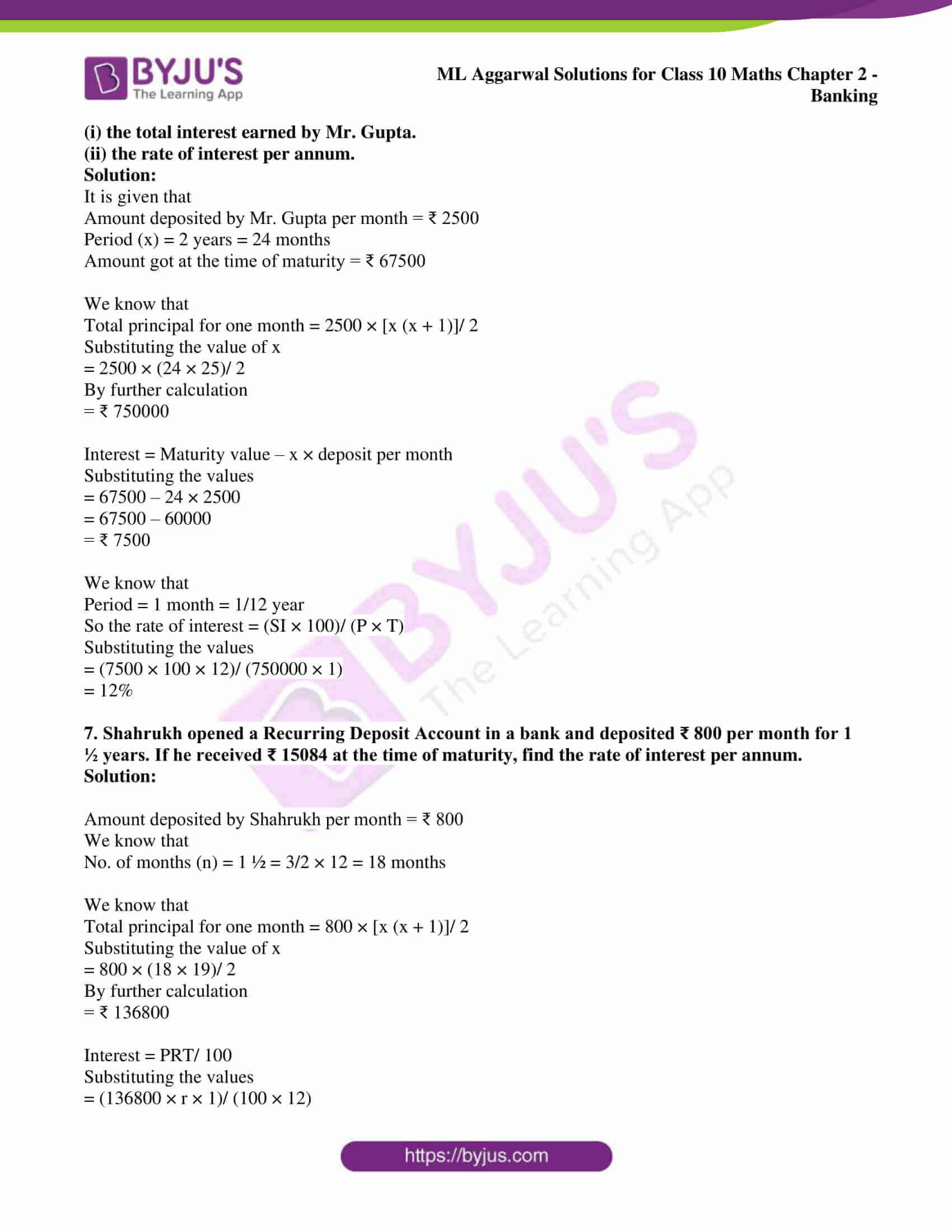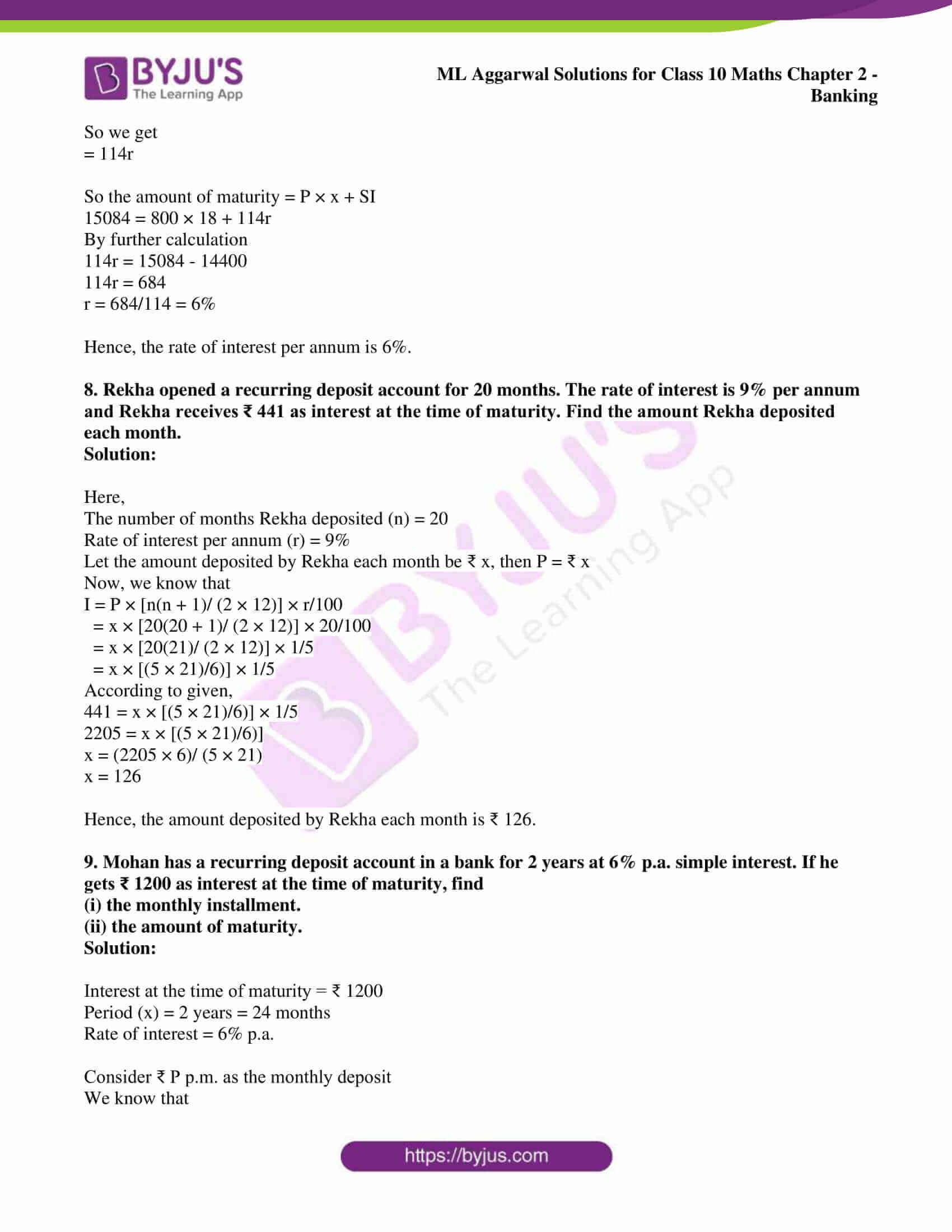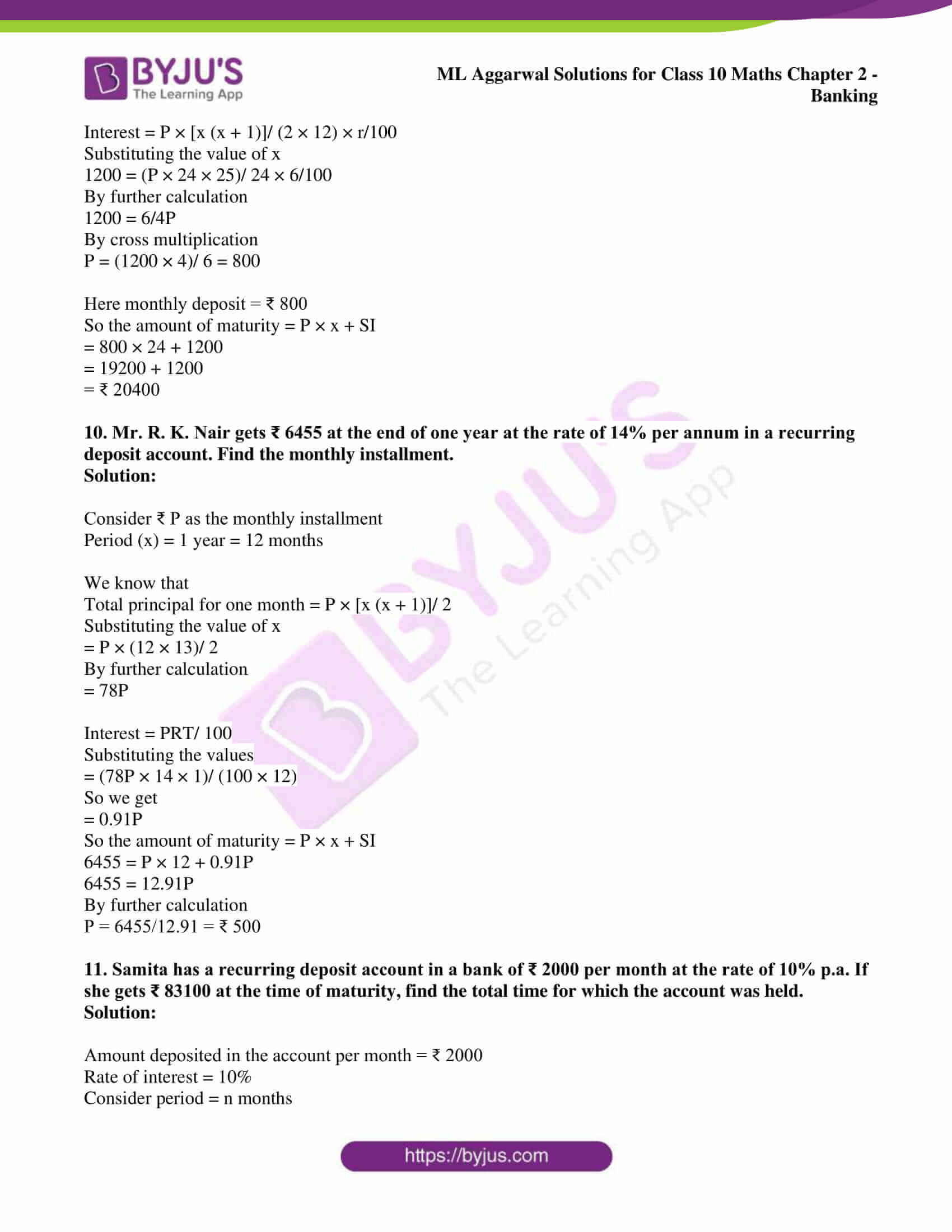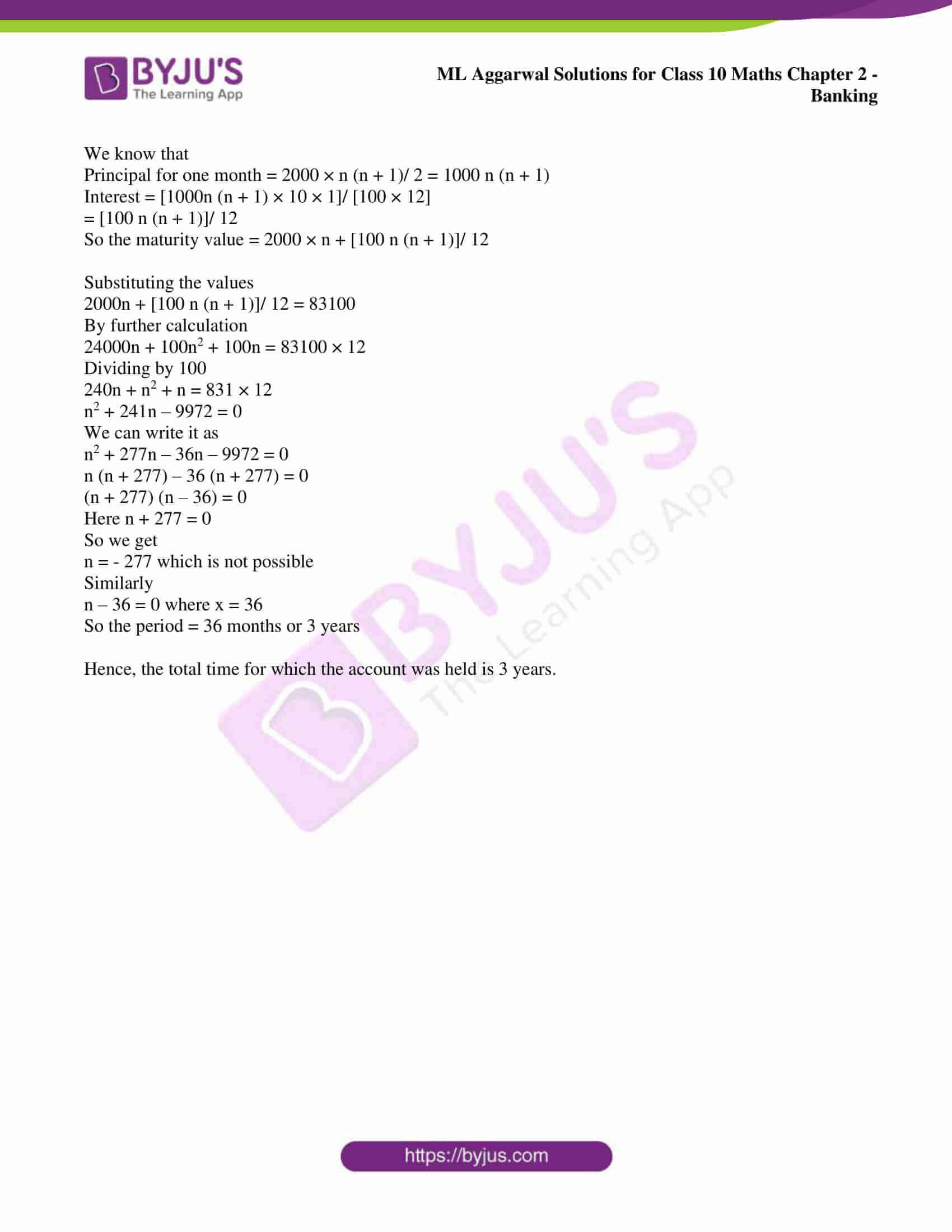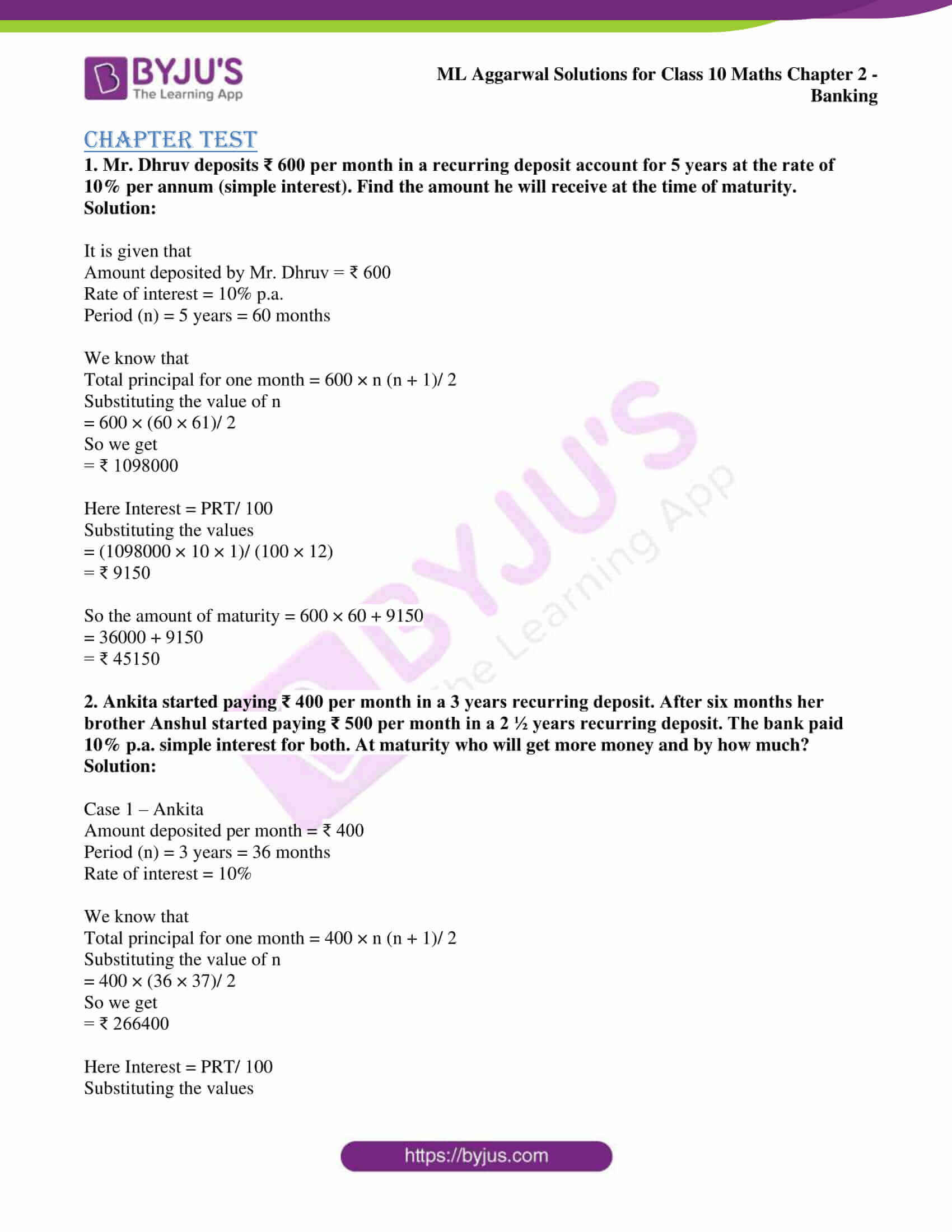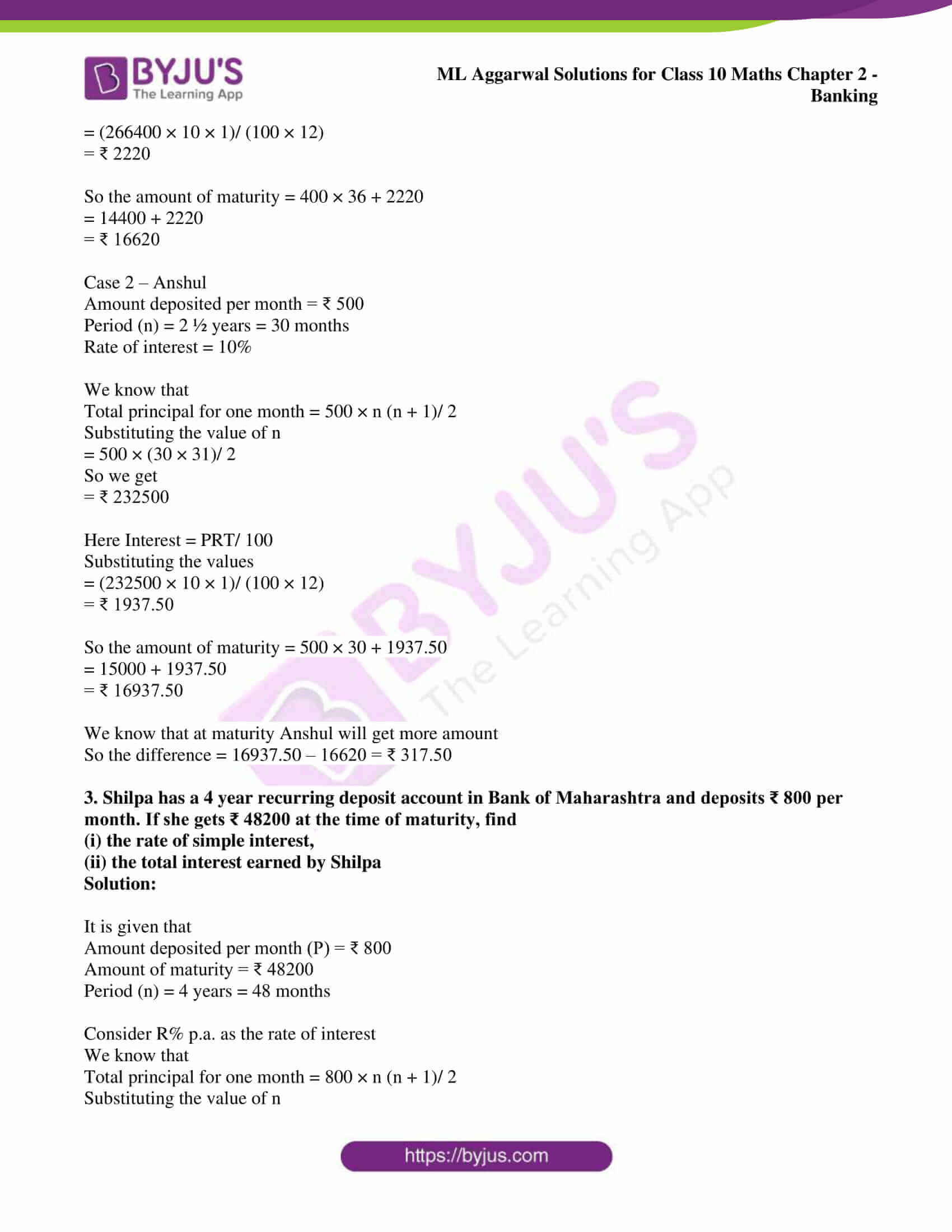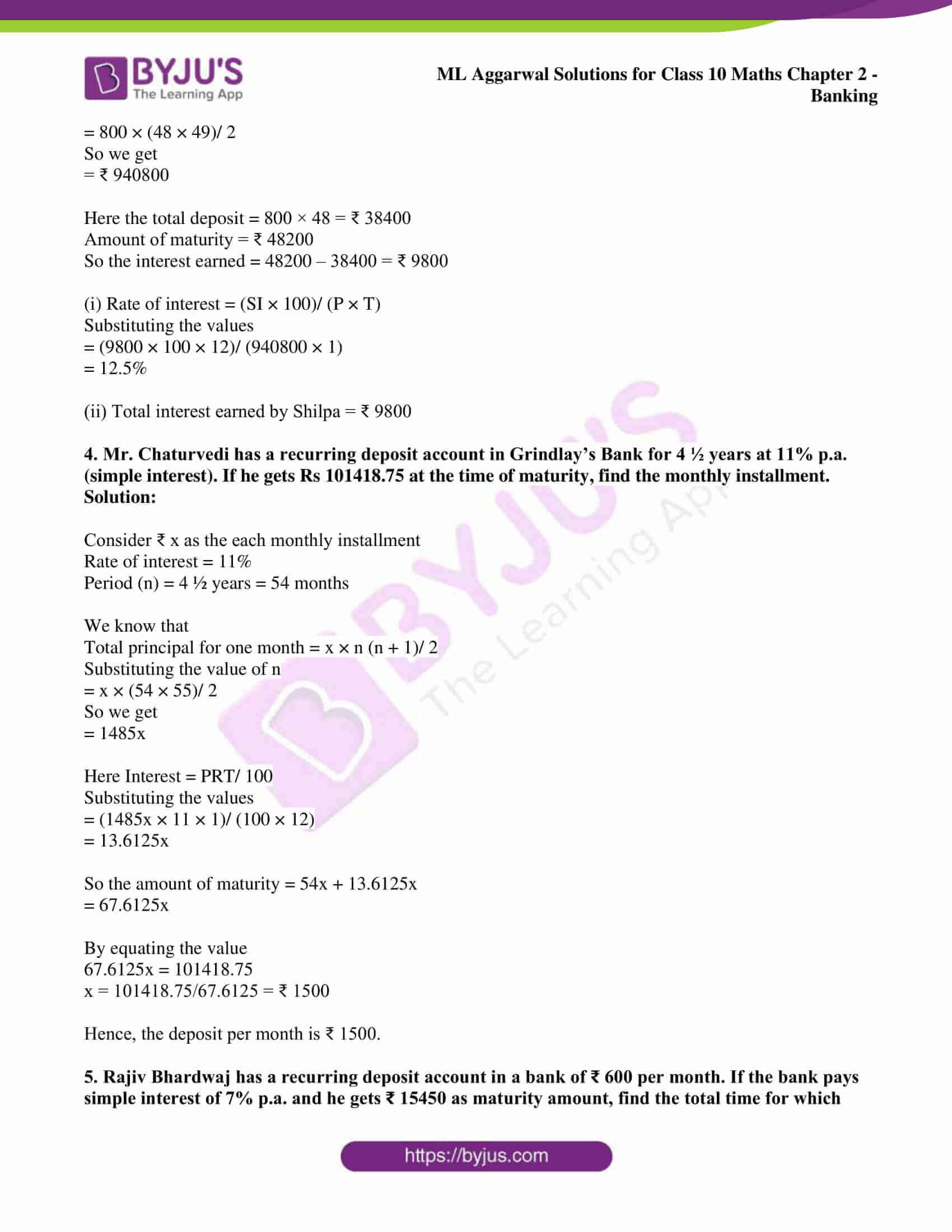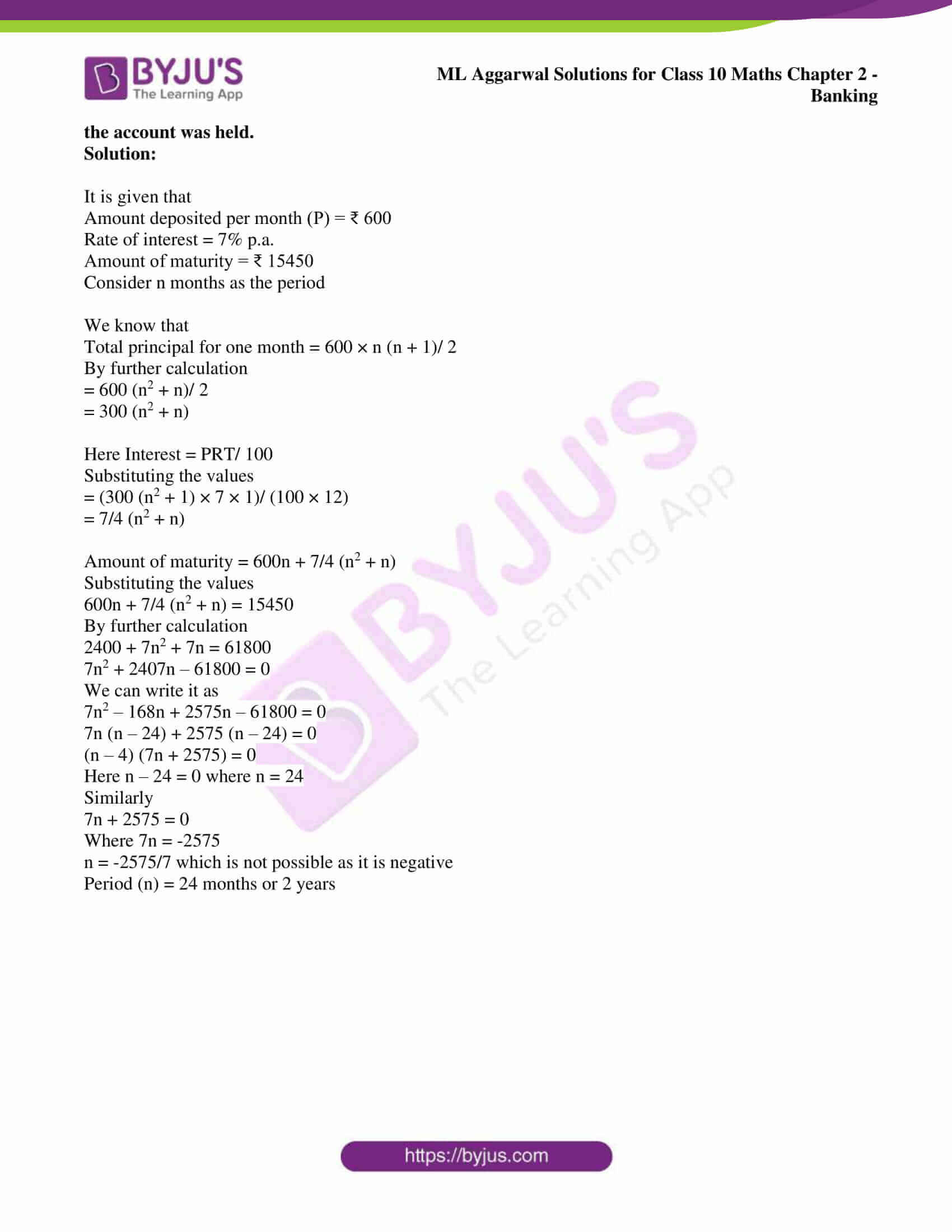## Access ML Aggarwal Solutions for Class 10 Maths Chapter 2: Banking

Exercise 2

1. Mrs. Goswami deposits ₹ 1000 every month in a recurring deposit account for 3 years at 8% interest per annum. Find the matured value.

Solution:

It is given that

Amount deposited by Mrs. Goswami = ₹ 1000

Rate of interest = 8% p.a.

Period (x) = 3 years = 36 months

We know that

Total principal for one month = 1000 × [x (x + 1)]/ 2

Substituting the value of x

= 1000 × (36 × 37)/ 2

By further calculation

= ₹ 666000

Interest = PRT/ 100

Substituting the values

= (666000 × 8 × 1)/ (100 × 12)

So we get

= ₹ 4440

So the amount of maturity = P × x + SI

= 1000 × 36 + 4440

= 36000 + 4440

= ₹ 40440

2. Sonia had a recurring deposit account in a bank and deposited ₹ 600 per month for 2 ½ years. If the rate of interest was 10% p.a., find the maturity value of this account.

Solution:

Its given that,

Amount deposited by Sonia per month = ₹ 600

Rate of interest (r) = 10% p.a.

Period (n) = 2 ½ years = 30 months

The interest earned during this period is calculated using the formula:

I = P × [n(n + 1)/ (2 × 12)] × r/100

I = 600 × [30(30 + 1)/ (2 × 12)] × 10/100

= 600 × [(30 × 31)/ (2 × 12)] × 1/10

= 60 × [(15 × 31)/ 12]

= 5 × 15 × 31

I = ₹ 2325

Maturity value (MV) = P × n + I

MV = ₹ (600 × 30 + 2325)

= ₹ (18000 + 2325)

= ₹ 20325

Hence, the maturity value of Sonia’s account will be ₹ 20325.

3. Kiran deposited ₹ 200 per month for 36 months in a bank’s recurring deposit account. If the banks pays interest at the rate of 11% per annum, find the amount she gets on maturity?

Solution:

It is given that

Amount deposited by Kiran = ₹ 200

Rate of interest = 11% p.a.

Period (x) = 36 months

So the amount deposited in 36 months = 200 × 36 = ₹ 7200

We know that

Total principal for one month = 200 × [x (x + 1)]/ 2

Substituting the value of x

= 200 × (36 × 37)/ 2

By further calculation

= ₹ 133200

Interest = PRT/ 100

Substituting the values

= (133200 × 11 × 1)/ (100 × 12)

So we get

= ₹ 1221

So the amount of maturity = P × x + SI

= 7200 + 1221

= ₹ 8421

4. Haneef has a cumulative bank account and deposits ₹ 600 per month for a period of 4 years. If he gets ₹ 5590 as interest at the time of maturity, find the rate of interest per annum.

Solution:

Interest at the time of maturity = ₹ 5880

Amount deposited by Haneef = ₹ 600

Period (x) = 4 years = 48 months

We know that

Total principal for one month = 600 × [x (x + 1)]/ 2

Substituting the value of x

= 600 × (48 × 49)/ 2

By further calculation

= ₹ 705600

Consider r% p.a. as the rate of interest

Interest = PRT/ 100

Substituting the values

5880 = (705600 × r × 1)/ (100 × 12)

So we get

5880 = 588r

By further calculation

r = 5880/588 = 10

Hence, the rate of interest = 10% p.a.

5. David opened a Recurring Deposit Account in a bank and deposited ₹ 300 per month for two years. If he received ₹ 7725 at the time of maturity, find the rate of interest per annum.

Solution:

It is given that

Amount deposited per month = ₹ 300

Period (x) = 2 years = 24 months

Amount received at the time of maturity = ₹ 7725

Consider R as the rate percent

We know that

Total principal for one month = 300 × [x (x + 1)]/ 2

Substituting the value of x

= 300 × (24 × 25)/ 2

By further calculation

= ₹ 90000

Interest = PRT/ 100

Substituting the values

= (90000 × R × 1)/ (100 × 12)

So we get

= 75R

So we get

300 × 24 + 75R = 7725

By further calculation

7200 + 75R = 7725

75R = 7725 – 7200 = 525

R = 525/75 = 7

Hence, the rate of interest is 7% p.a.

6. Mr. Gupta opened a recurring deposit account in a bank. He deposited ₹ 2500 per month for two years. At the time of maturity he got ₹ 67500. Find:

(i) the total interest earned by Mr. Gupta.

(ii) the rate of interest per annum.

Solution:

It is given that

Amount deposited by Mr. Gupta per month = ₹ 2500

Period (x) = 2 years = 24 months

Amount got at the time of maturity = ₹ 67500

We know that

Total principal for one month = 2500 × [x (x + 1)]/ 2

Substituting the value of x

= 2500 × (24 × 25)/ 2

By further calculation

= ₹ 750000

Interest = Maturity value – x × deposit per month

Substituting the values

= 67500 – 24 × 2500

= 67500 – 60000

= ₹ 7500

We know that

Period = 1 month = 1/12 year

So the rate of interest = (SI × 100)/ (P × T)

Substituting the values

= (7500 × 100 × 12)/ (750000 × 1)

= 12%

7. Shahrukh opened a Recurring Deposit Account in a bank and deposited ₹ 800 per month for 1 ½ years. If he received ₹ 15084 at the time of maturity, find the rate of interest per annum.

Solution:

Amount deposited by Shahrukh per month = ₹ 800

We know that

No. of months (n) = 1 ½ = 3/2 × 12 = 18 months

We know that

Total principal for one month = 800 × [x (x + 1)]/ 2

Substituting the value of x

= 800 × (18 × 19)/ 2

By further calculation

= ₹ 136800

Interest = PRT/ 100

Substituting the values

= (136800 × r × 1)/ (100 × 12)

So we get

= 114r

So the amount of maturity = P × x + SI

15084 = 800 × 18 + 114r

By further calculation

114r = 15084 – 14400

114r = 684

r = 684/114 = 6%

Hence, the rate of interest per annum is 6%.

8. Rekha opened a recurring deposit account for 20 months. The rate of interest is 9% per annum and Rekha receives ₹ 441 as interest at the time of maturity. Find the amount Rekha deposited each month.

Solution:

Here,

The number of months Rekha deposited (n) = 20

Rate of interest per annum (r) = 9%

Let the amount deposited by Rekha each month be ₹ x, then P = ₹ x

Now, we know that

I = P × [n(n + 1)/ (2 × 12)] × r/100

= x × [20(20 + 1)/ (2 × 12)] × 20/100

= x × [20(21)/ (2 × 12)] × 1/5

= x × [(5 × 21)/6)] × 1/5

According to given,

441 = x × [(5 × 21)/6)] × 1/5

2205 = x × [(5 × 21)/6)]

x = (2205 × 6)/ (5 × 21)

x = 126

Hence, the amount deposited by Rekha each month is ₹ 126.

9. Mohan has a recurring deposit account in a bank for 2 years at 6% p.a. simple interest. If he gets ₹ 1200 as interest at the time of maturity, find

(i) the monthly installment.

(ii) the amount of maturity.

Solution:

Interest at the time of maturity = ₹ 1200

Period (x) = 2 years = 24 months

Rate of interest = 6% p.a.

Consider ₹ P p.m. as the monthly deposit

We know that

Interest = P × [x (x + 1)]/ (2 × 12) × r/100

Substituting the value of x

1200 = (P × 24 × 25)/ 24 × 6/100

By further calculation

1200 = 6/4P

By cross multiplication

P = (1200 × 4)/ 6 = 800

Here monthly deposit = ₹ 800

So the amount of maturity = P × x + SI

= 800 × 24 + 1200

= 19200 + 1200

= ₹ 20400

10. Mr. R. K. Nair gets ₹ 6455 at the end of one year at the rate of 14% per annum in a recurring deposit account. Find the monthly installment.

Solution:

Consider ₹ P as the monthly installment

Period (x) = 1 year = 12 months

We know that

Total principal for one month = P × [x (x + 1)]/ 2

Substituting the value of x

= P × (12 × 13)/ 2

By further calculation

= 78P

Interest = PRT/ 100

Substituting the values

= (78P × 14 × 1)/ (100 × 12)

So we get

= 0.91P

So the amount of maturity = P × x + SI

6455 = P × 12 + 0.91P

6455 = 12.91P

By further calculation

P = 6455/12.91 = ₹ 500

11. Samita has a recurring deposit account in a bank of ₹ 2000 per month at the rate of 10% p.a. If she gets ₹ 83100 at the time of maturity, find the total time for which the account was held.

Solution:

Amount deposited in the account per month = ₹ 2000

Rate of interest = 10%

Consider period = n months

We know that

Principal for one month = 2000 × n (n + 1)/ 2 = 1000 n (n + 1)

Interest = [1000n (n + 1) × 10 × 1]/ [100 × 12]

= [100 n (n + 1)]/ 12

So the maturity value = 2000 × n + [100 n (n + 1)]/ 12

Substituting the values

2000n + [100 n (n + 1)]/ 12 = 83100

By further calculation

24000n + 100n2 + 100n = 83100 × 12

Dividing by 100

240n + n2 + n = 831 × 12

n2 + 241n – 9972 = 0

We can write it as

n2 + 277n – 36n – 9972 = 0

n (n + 277) – 36 (n + 277) = 0

(n + 277) (n – 36) = 0

Here n + 277 = 0

So we get

n = – 277 which is not possible

Similarly

n – 36 = 0 where x = 36

So the period = 36 months or 3 years

Hence, the total time for which the account was held is 3 years.

Chapter Test

1. Mr. Dhruv deposits ₹ 600 per month in a recurring deposit account for 5 years at the rate of 10% per annum (simple interest). Find the amount he will receive at the time of maturity.

Solution:

It is given that

Amount deposited by Mr. Dhruv = ₹ 600

Rate of interest = 10% p.a.

Period (n) = 5 years = 60 months

We know that

Total principal for one month = 600 × n (n + 1)/ 2

Substituting the value of n

= 600 × (60 × 61)/ 2

So we get

= ₹ 1098000

Here Interest = PRT/ 100

Substituting the values

= (1098000 × 10 × 1)/ (100 × 12)

= ₹ 9150

So the amount of maturity = 600 × 60 + 9150

= 36000 + 9150

= ₹ 45150

2. Ankita started paying ₹ 400 per month in a 3 years recurring deposit. After six months her brother Anshul started paying ₹ 500 per month in a 2 ½ years recurring deposit. The bank paid 10% p.a. simple interest for both. At maturity who will get more money and by how much?

Solution:

Case 1 – Ankita

Amount deposited per month = ₹ 400

Period (n) = 3 years = 36 months

Rate of interest = 10%

We know that

Total principal for one month = 400 × n (n + 1)/ 2

Substituting the value of n

= 400 × (36 × 37)/ 2

So we get

= ₹ 266400

Here Interest = PRT/ 100

Substituting the values

= (266400 × 10 × 1)/ (100 × 12)

= ₹ 2220

So the amount of maturity = 400 × 36 + 2220

= 14400 + 2220

= ₹ 16620

Case 2 – Anshul

Amount deposited per month = ₹ 500

Period (n) = 2 ½ years = 30 months

Rate of interest = 10%

We know that

Total principal for one month = 500 × n (n + 1)/ 2

Substituting the value of n

= 500 × (30 × 31)/ 2

So we get

= ₹ 232500

Here Interest = PRT/ 100

Substituting the values

= (232500 × 10 × 1)/ (100 × 12)

= ₹ 1937.50

So the amount of maturity = 500 × 30 + 1937.50

= 15000 + 1937.50

= ₹ 16937.50

We know that at maturity Anshul will get more amount

So the difference = 16937.50 – 16620 = ₹ 317.50

3. Shilpa has a 4 year recurring deposit account in Bank of Maharashtra and deposits ₹ 800 per month. If she gets ₹ 48200 at the time of maturity, find

(i) the rate of simple interest,

(ii) the total interest earned by Shilpa

Solution:

It is given that

Amount deposited per month (P) = ₹ 800

Amount of maturity = ₹ 48200

Period (n) = 4 years = 48 months

Consider R% p.a. as the rate of interest

We know that

Total principal for one month = 800 × n (n + 1)/ 2

Substituting the value of n

= 800 × (48 × 49)/ 2

So we get

= ₹ 940800

Here the total deposit = 800 × 48 = ₹ 38400

Amount of maturity = ₹ 48200

So the interest earned = 48200 – 38400 = ₹ 9800

(i) Rate of interest = (SI × 100)/ (P × T)

Substituting the values

= (9800 × 100 × 12)/ (940800 × 1)

= 12.5%

(ii) Total interest earned by Shilpa = ₹ 9800

4. Mr. Chaturvedi has a recurring deposit account in Grindlay’s Bank for 4 ½ years at 11% p.a. (simple interest). If he gets Rs 101418.75 at the time of maturity, find the monthly installment.

Solution:

Consider ₹ x as the each monthly installment

Rate of interest = 11%

Period (n) = 4 ½ years = 54 months

We know that

Total principal for one month = x × n (n + 1)/ 2

Substituting the value of n

= x × (54 × 55)/ 2

So we get

= 1485x

Here Interest = PRT/ 100

Substituting the values

= (1485x × 11 × 1)/ (100 × 12)

= 13.6125x

So the amount of maturity = 54x + 13.6125x

= 67.6125x

By equating the value

67.6125x = 101418.75

x = 101418.75/67.6125 = ₹ 1500

Hence, the deposit per month is ₹ 1500.

5. Rajiv Bhardwaj has a recurring deposit account in a bank of ₹ 600 per month. If the bank pays simple interest of 7% p.a. and he gets ₹ 15450 as maturity amount, find the total time for which the account was held.

Solution:

It is given that

Amount deposited per month (P) = ₹ 600

Rate of interest = 7% p.a.

Amount of maturity = ₹ 15450

Consider n months as the period

We know that

Total principal for one month = 600 × n (n + 1)/ 2

By further calculation

= 600 (n2 + n)/ 2

= 300 (n2 + n)

Here Interest = PRT/ 100

Substituting the values

= (300 (n2 + 1) × 7 × 1)/ (100 × 12)

= 7/4 (n2 + n)

Amount of maturity = 600n + 7/4 (n2 + n)

Substituting the values

600n + 7/4 (n2 + n) = 15450

By further calculation

2400 + 7n2 + 7n = 61800

7n2 + 2407n – 61800 = 0

We can write it as

7n2 – 168n + 2575n – 61800 = 0

7n (n – 24) + 2575 (n – 24) = 0

(n – 4) (7n + 2575) = 0

Here n – 24 = 0 where n = 24

Similarly

7n + 2575 = 0

Where 7n = -2575

n = -2575/7 which is not possible as it is negative

Period (n) = 24 months or 2 years